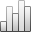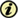Acta Stereologica

0351-580XMore Statisticssince 05 December 2013 :
View(s): 232 (4 ULiège)
Michel Schmitt

### Propagation function: towards constant time algorithms

(Volume 13 (1994) — Number 1 - Proceedings of the sixth European congress for stereology - Part two - May 1994)
Article#### Abstract

Let Pn be the regular n-sided polygon inscribed in a circle of radius 1 in the plane. The distance from x to y induced by Pn is the smallest size of the homothety of Pn centered at x and containing y. On X, simply connected planar set, the propagation functionis defined by(x) = supyX(x,y) where(x,y) is the geodesic distance in X, that is the lower bound of the length (induced by Pn) of the paths entirely lying inside X and linking x to y. Efficient algorithms forare based on the following remark: the farthest points to any x in X may have only a few possible locations Y. In this paper, it is shown that, as in the convex case, there exists a set Y with at most n elements, such that(x) = supyY(x,y). In the case of the square lattice equipped with the 4-connectivity distance, this theorem leads to an algorithm computing the propagation function by means of at most 7 geodesic balls, whatever the shape of X.

Keywords : digital metrics, geodesic distance, propagation function